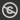Science, Maths & Technology
Free course

# Point estimation## Course reviews

This free course looks at point estimation, that is, the estimation of the value of the parameter of a statistical model by a single number, a point estimate for the parameter. Section 1 develops some aspects of maximum likelihood estimation. In particular, you will find out how to obtain the maximum likelihood estimator of an unknown parameter, using calculus. You will need to do lots of differentiation in this section. Section 2 introduces a number of important properties of point estimation.

## Course learning outcomes

After studying this course, you should be able to:

• calculate the maximum likelihood estimator using calculus
• work with the log-likelihood instead of the likelihood itself
• understand that maximum likelihood estimation of a function of a parameter is equivalent to taking that function of the maximum likelihood estimator
• define and calculate the bias of an estimator and choose between unbiased estimators on the basis of their variances
• understand and calculate the Cramèr-Rao lower bound for the variance of any unbiased estimator.

First Published: 12/03/2018

Updated: 09/04/2019

Skip Rate and Review